Machine Learning is the applied science of making computers work without being explicitly programmed. Machine Learning keeps on innovating every aspect of businesses and has been shaping up the future even more powerfully now. Starting from housekeeping to new drug discovery, machine learning has revolutionized the way things were done earlier. Opportunities are immense in the high-paying field of machine learning and companies across different industries are now employing a candidate with relevant subject knowledge and expertise. It is a broad field and you cannot predict what type of specific Machine Learning interview questions will be asked in a job interview. Most of these questions will focus on the open job position the employer is trying to fill. Take a look at some of the most commonly asked Machine Learning interview questions.

## Top Machine Learning Interview Questions & Answers

### Q1. What are some of the most commonly used Machine Learning algorithms?

Ans. Some of the popular Machine Learning algorithms are –

• Linear Regression
• Logistic RegressionDecision Tree
SVM
• Naive Bayes
• kNN
• K-Means
• Random Forest
• Dimensionality Reduction Algorithms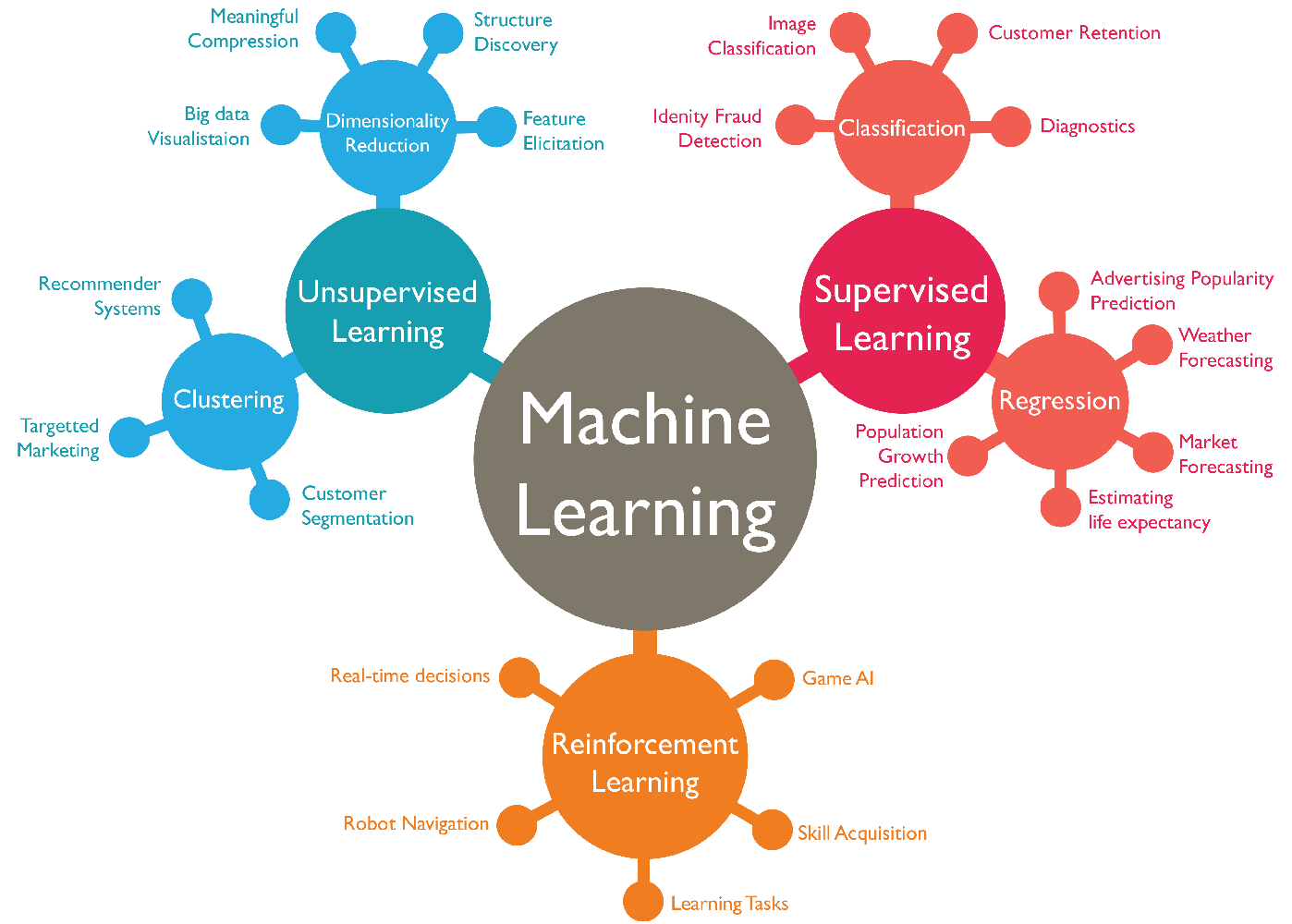Image – Machine Learning Algorithms (Source)

### Q2. What is the difference between Data Mining and Machine learning?

Ans: Machine Learning is about the study, design, and development of the algorithms that make computers work without being explicitly programmed.

Data Mining is a process wherein the unstructured data tries to extract knowledge or unknown interesting patterns, using Machine Learning algorithms.

### Q3. What are Convolutional neural networks?

Ans. Convolutional Neural Networks are a type of Artificial Neural Networks with supervised learning that processes its layers by imitating the visual cortex of the human eye to identify different characteristics in the inputs that ultimately make it able to identify objects and “see”.

Here “neurons” correspond to receptive fields in a very similar way to neurons in the primary visual cortex (V1) of a human brain. Convolutional neural networks consist of multiple specialized hidden layers of convolutional filters of one or more dimensions. After each layer, a function is added to perform non-linear causal mapping and recognize complex shapes such as a face or the silhouette of a human or an animal.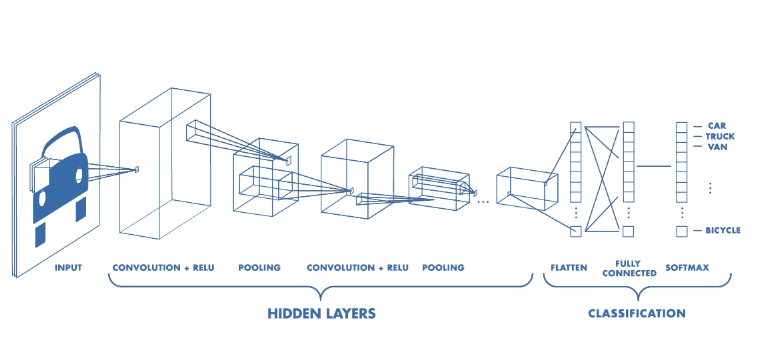Image – Basic Convolutional Neural Network (Source)

### Q4. What are the different types of Convolutional Neural Networks?

Ans. There are five types of Convolutional Neural Networks –

• LeNet
• AlexNet
• VGG-16 Net
• ResNet
• Inception Net

LeNet – LeNet is one of the oldest convolution neural networks designed to classify handwritten digits from 0–9, of the MNIST Dataset. It comprises 7 layers, which includes two sets of both convolutional and average pooling layers, a flattening convolutional layer, two fully connected layers, and a softmax classifier.Image – LeNet Architecture (Original image published in [LeCun et al., 1998]) (Source)

AlexNet – AlexNet is similar to LeNet but has a deeper architecture with 8 layers and added filters. The layers include convolutional layers, max pooling, dropout, data augmentation, ReLU, and SGD.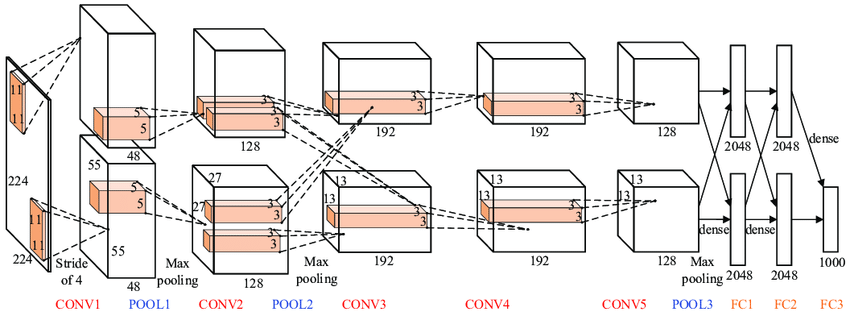Image – AlexNet Architecture (Source)

VGG-16 Net– VGG-16 Net is a 16 layers deep convolutional neural network. It has many layers but the architecture is very simple and is among the most preferred type of convolutional neural networks among developers. VGG-16 Net has 140 million parameters to handle, thus ensuring the most efficient output.Image – VGG-16 Net Architecture (Source)

ResNet – ResNet or Residual networks are over 100 layers of deep networks. These networks learn residual functions with reference to the layer inputs and skip connections solve the problem of vanishing gradient in deep neural networks. The below image describes normal deep networks vs networks with skip connections, showing the difference in outputs.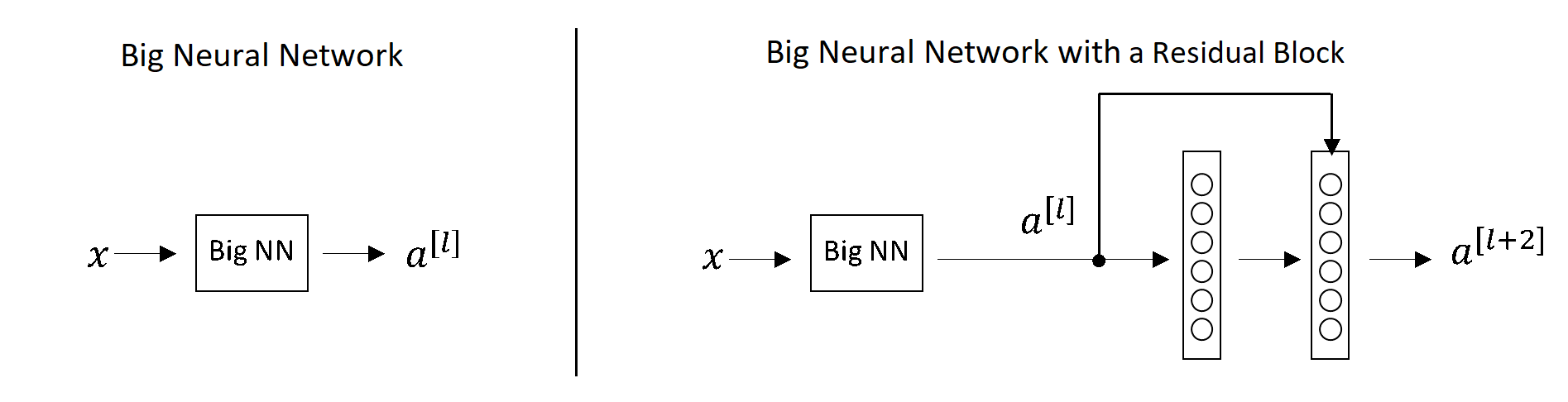Image – Normal Deep Networks vs Networks with skip connections (Source)

Inception Net – Inception Net or GoogleLeNet has a 27 layers deep architecture and is designed to tackle loss of data. It uses input data to compute multiple different conversions in parallel, concatenating them in a single output.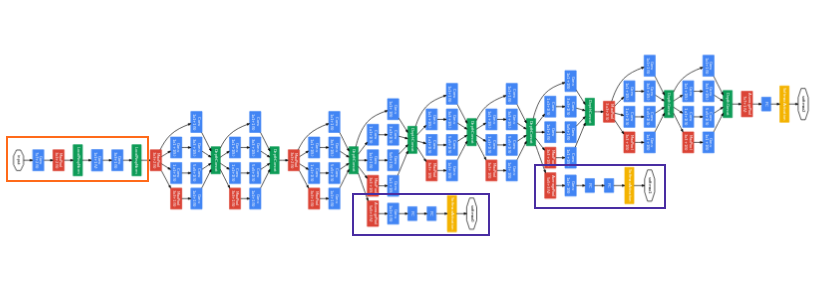Image – Inception Net or GoogLeNet – Orange box – Stem; Purple boxes – Auxiliary classifiers; Wide parts – Inception modules (Source)

### Q5. What do you understand by ensemble learning?

Ans: Ensemble learning is a machine learning technique that uses various base models such as classifiers or experts to produce an optimal predictive model. To solve any computational program, such models are strategically generated and combined. The ensemble is a supervised learning algorithm, as it can be trained and used to make predictions.

### Q6. What are the different stages of building a model in Machine Learning?

Ans: It is one of the most important machine learning interview questions.

There are four stages of building a model in Machine Learning –

• Manage data
• Train models
• Evaluate models
• Deploy models

### Q7. Can you use machine learning for time series analysis?

Ans. Yes, it can be used but it depends on the applications. The predictive models based on machine learning have wide applicability across time series projects. These models help in facilitating the predictive distribution of time and resources. The most widely applied machine learning methods for time series forecasting projects are –

• Multi-Layer Perceptron (MLP)
• Recurrent Neural Network (RNN)
• Long Short-Term Memory (LSTM)### Q8. What does NLP stand for?

Ans. NLP stands for Natural Language Processing. It is a branch of artificial intelligence that gives machines the ability to read and understand human languages.

### Q9. Name the paradigms of ensemble methods.

Ans: There are two paradigms of ensemble methods, which are –

• Sequential ensemble methods
• Parallel ensemble methods

To learn about data science, read our blog on – What is data science?

### Q10. What is regularization?

Ans: Regularization is a technique to improve the validation score. Most of the time, it is achieved by reducing the training score.

### Q11. What are the full forms of PCA, KPCA, and ICA, and what is their use?

Ans: PCA – Principal Components Analysis

KPCA – Kernel-based Principal Component Analysis

ICA – Independent Component Analysis

These are important feature extraction techniques, which are majorly used for dimensionality reduction.

### Q12. Name the components of relational evaluation techniques.

Ans: The main components of relational evaluation techniques are –

• Data Acquisition
• Ground Truth Acquisition
• Cross-Validation Technique
• Query Type
• Scoring Metric
• Significance Test

### Q13. What is a Confusion Matrix?

Ans: Also known as the error matrix, a confusion matrix is a table that summarizes the performance of a classification algorithm.

### Q14. What is a ROC curve?

Ans: It is a Receiver Operating Characteristic curve, a fundamental tool for diagnostic test evaluation. ROC curve is a plot of Sensitivity against Specificity for probable cut-off points of a diagnostic test. It is the graphical representation of the contrast between true positive rates and the false positive rate at different thresholds.

### Q15. Can you name some libraries in Python used for Data Analysis and Scientific Computations?

Ans: Python is among the most discussed topics in machine learning interview questions.

Some of the key Python libraries used in Data Analysis include –

• Bokeh
• Matplotlib
• NumPy
• Pandas
• SciKit
• SciPy
• Seaborn

### Q16. Cite the difference between supervised and unsupervised machine learning.

Ans: Supervised learning is all about training labeled data for tasks like data classification, while unsupervised learning does not require explicitly labeling data.

### Q17. Name different methods to solve Sequential Supervised Learning problems.

Ans: Some of the most popular methods to solve Sequential Supervised Learning problems include –

• Sliding-window methods
• Recurrent sliding windows
• Hidden Markov models
• Maximum entropy Markov models
• Conditional random fields
• Graph transformer networks

### Q18. What is the use of Box-Cox transformation?

Ans: The Box-Cox transformation is a generalized “power transformation” that ensures normal data transformation and distribution. It is used to eliminate heteroscedasticity.

### Q19. What is a Fourier transform?

Ans: It is a generic method to breaks a waveform into an alternate representation, mainly characterized by sine and cosines.

### Q20. What is PAC Learning?

Ans: It is an abbreviation for Probably Approximately Correct. This learning framework analyzes learning algorithms and statistical efficiency.

### Q21. What are the different machine learning approaches?

Ans. The different machine learning approaches are –

• Concept Vs. Classification Learning
• Symbolic Vs. Statistical Learning
• Inductive Vs. Analytical Learning

### Q22. What is the main difference between overfitting and underfitting?

Ans: It is among very commonly asked machine learning interview questions.

Overfitting – In overfitting, a statistical model describes any random error or noise, and occurs when a model is super complicated. An overfit model has poor predictive performance as it overreacts to minor fluctuations in training data.
Underfitting – In underfitting, a statistical model is unable to capture the underlying data trend. This type of model also shows poor predictive performance.

### Q23. What is Gradient Descent?

Ans: Gradient Descent is a popular algorithm used for training Machine Learning models. It is also used to find the values of parameters of a function (f) to minimize a cost function.

### Q24. What is the difference between Causation and Correlation?

Ans: Causation denotes any causal relationship between two events and represents its cause and effects.
Correlation determines the relationship between two or more variables.
Causation necessarily denotes the presence of correlation, but correlation does not necessarily denote causation.

### Q25. What is the difference between a Validation Set and a Test Set?

Ans: The validation set is used to minimize overfitting. This is used in parameter selection, which means that it helps to verify any accuracy improvement over the training data set.

Test Set is used to test and evaluate the performance of a trained Machine Learning model.

### Q26. What is a Boltzmann Machine?

Ans: Boltzmann Machines have a simple learning algorithm that helps to discover exciting features in training data. These were among the first neural networks to learn internal representations and are capable of solving severe combinatory problems.

### Q27. What are Recommender Systems?

Ans: Recommender systems are information filtering systems that predict which products will attract customers, but these systems are not ideal for every business situation. These systems are used in movies, news, research articles, products, etc. These systems are content and collaborative filtering-based.

### Q28.  What is Deep Learning?

Ans: Deep Learning is an artificial intelligence function used in decision making. It is among the most important functions of machine learning and among the most commonly asked machine learning interview questions.

Deep Learning imitates the human brain’s functioning to process the data and create the patterns used in decision-making. Deep learning is a key technology behind automated driving, automated machine translation, automated game playing, object classification in photographs, and automated handwriting generation, among others.

### Q29. What are imbalanced datasets?

Ans: Imbalanced datasets refer to the different numbers of data points available for different classes.

### Q30. How would you handle imbalanced datasets?

Ans: We can handle imbalanced datasets in the following ways –

Oversampling/Undersampling – We can use oversampling or undersampling instead of sampling with a uniform distribution from the training dataset. This will help to see a more balanced dataset.

Data augmentation – We can modify the existing data in a controlled way by adding data in the less frequent categories.

Use of appropriate metrics – Usage of metrics like precision, recall, and F-score can help to describe the model accuracy in a better way if an imbalanced dataset is being used.

### Q31.  What is Pattern Recognition?

Ans: Pattern recognition is the process of data classification by recognizing patterns and data regularities. This methodology involves the use of machine learning algorithms.

### Q32.  Where can you use Pattern Recognition?

Ans: Pattern Recognition can be used in

• Bio-Informatics
• Computer Vision
• Data Mining
• Informal Retrieval
• Statistics
• Speech Recognition

### Q33. What is Data augmentation? Can you give an example?

Ans: Data augmentation is a machine learning strategy that enables the users to increase the data diversity for training models remarkably from internal and external sources within an enterprise. This does not require any new data collection.

Modification in images is one of the most helpful examples of data augmentation. We can easily perform the following activities on an image and modify it –

• Resizing the image
• Flipping it horizontally or vertically
• Deforming
• Modifying colors

### Q34. Mention the differences between Type I and Type II errors.

Ans: The most significant differences between Type I and Type II errors are –

 Type I Error Type II Error False-positive error False-negative error Claims something when nothing has happened Claims nothing when something has happened It is the probability of rejecting a true null hypothesis It is the probability of failing to reject a false null hypothesis

### Q35. How will you perform static analysis in a Python application?

Ans: PyChecker can be helpful as a static analyzer to identify the bugs in the Python project. This also helps to find out the complexity-related bugs. Pylint is another tool that is helpful in checking if the Python module is at par with the coding standards.

### Q36. What is Genetic Programming?

Ans: Genetic Programming is a type of Evolutionary Algorithm (EA). It can be used to solve problems across different fields, including optimization, automatic programming, and machine learning. Genetic Programming is inspired by biological evolution. This system implements algorithms that use random mutation, crossover, fitness functions, and multiple generations of evolution, which altogether contribute to solving user-defined tasks.

### Q37. What are the different types of Genetic Programming?

Ans: Different types of Genetic Programming are –

• Cartesian Genetic Programming (CGP)
• Extended Compact Genetic Programming (ECGP)
• Genetic Improvement of Software for Multiple Objectives (GISMO)
• Grammatical Evolution
• Linear Genetic Programming (LGP)
• Probabilistic Incremental Program Evolution (PIPE)
• Stack-based Genetic Programming
• Strongly Typed Genetic Programming (STGP)
• Tree-based Genetic Programming

### Q38. What is the Model Selection?

Ans: It is one of the most important machine learning interview questions.

Model Selection refers to a process of selecting models from different mathematical models for describing the same data set. The model selection has its applications across various fields, including statistics, machine learning as well as data mining.

### Q39. Which classification methods can be handled by Support Vector Machines?

Ans: SVMs can handle two classification methods –

• Combining binary classifiers
• Modifying binary to incorporate multiclass learning

### Q40. In how many groups can SVM models be classified?

Ans: SVM models are classified into four distinct groups:

• Classification SVM Type 1 (also called C-SVM classification)
• Classification SVM Type 2 (also called nu-SVM classification)
• Regression SVM Type 1 (also called epsilon-SVM regression)
• Regression SVM Type 2 (also called nu-SVM regression)

### Q41. High variance in data – is it good or bad?

Ans: It is bad. Higher variance in the data suggests that the spread of data is bigger and the dataset is not presenting a very accurate or representative picture of the relationship between the inputs and predicted output.

### Q42. If your dataset has the issue of high variance, how would you handle it?

Ans: We can use a bagging algorithm to handle the high variance in datasets. These algorithms split the data into subgroups with sampling replicated from random data. After the data is split, we can use random data to create rules using a training algorithm. We can then use the polling technique to combine all the predicted outcomes of the dataset.

### Q43. What knowledge do you need to have to extract the predicted information from the raw data?

Ans. To extract the predicted information from the raw data, one must have a good understanding of mathematics, statistics, computer science, machine learning, data visualization, cluster analysis, and data modeling.

### Q44. What is logistic regression?

Ans. Logistic regression is a statistical technique used to predict a binary result that is zero or one, or a yes or a no.

### Q45. Why is data cleansing important in data analysis?

Ans. Data is accumulated from a variety of sources. It is important to ensure that the data collected is good enough for analysis. Data cleaning or erasure ensures that data is complete and accurate, and does not contain redundant or irrelevant components.

### Q45. What does the A/B test aim to accomplish?

Ans. It is a statistical hypothesis test used to detect any changes to the website so that measures can be taken to maximize the possibility of the desired result.

### Q46. Python or R – Which is the best for machine learning?

Ans. In machine learning projects, both R and Python come with their own advantages. However, Python is more useful in data manipulation and repetitive tasks, making it the right choice if you plan to build a digital product based on machine learning. Moreover, to develop a tool for ad-hoc analysis at an early stage of the project, R is more suitable.

### Q47. What is TF / IDF vectorization?

Ans. TF-IDF stands for Reverse Document Frequency. It is a numerical statistic is used to determine the importance of a word in a document of a collection or corpus.

### Q48. What are tensioners?

Ans. Tensors are similar to matrices in programming languages, but here they are larger. Tensors can be considered as a generalization of matrices that form a matrix of n dimensions. TensorFlow provides methods that can be used to easily create tensor functions and calculate their derivatives. This is what distinguishes tensors from NumPy matrices.

### Q49. What are the benefits of using TensorFlow?

Ans. TensorFlow has numerous advantages, which is why it is the most widely used framework for machine learning. Some of which include –

• Platform independence
• GPU use for distributed computing
• Self-differentiation capacity
• Open source and a great community
• Highly customizable according to requirements
• Support for asynchronous calculations

### Q50. Are there any limitations to using TensorFlow?

Ans. Although TensorFlow offers numerous benefits, it has a caveat or two in current versions:

• No support for OpenCL (Open Computing Language) yet
• GPU memory conflicts when used with Theano
• It can be overwhelming for beginners to start

### Q51. Can we capture the correlation between continuous and categorical variables?

Ans: Yes, we can establish the correlation between continuous and categorical variables by using the Analysis of Covariance or ANCOVA technique. ANCOVA controls the effects of selected other continuous variables, which co-vary with the dependent.

### Q52. What is selection bias?

Ans: A statistical error that leads to a bias in the sampling portion of an experiment is called selection bias. If the selection bias remains unidentified, it may lead to a wrong conclusion.

### Q53. What is a Hash Table?

Ans: A Hash Table is a data structure that produces an associative array, and is used for database indexing.

————————————————————————————————————–

If you have recently completed a professional course/certification, click here to submit a review and get FREE certification highlighter worth Rs. 500.

5.00 avg. rating (98% score) - 4 votes